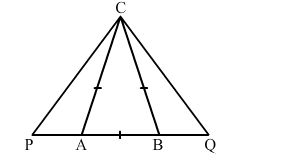# In an isosceles ∆ ABC, the base AB is produced both ways to P and Q, such that AP × BQ = AC2.

Question:

In an isosceles ∆ ABC, the base AB is produced both ways to P and Q, such that AP × BQ = AC2.
Prove that ∆ ACP ∼ ∆ BCQ.Solution:

Disclaimer: It should be $\triangle A P C \sim \triangle B C Q$ instead of $\triangle A C P \sim \triangle B C Q$

It is given that $\triangle \mathrm{ABC}$ is an isosceles triangle.

Therefore,
CA = CB

$\Rightarrow \angle C A B=\angle C B A$

$\Rightarrow 180^{\circ}-\angle C A B=180^{\circ}-\angle C B A$

$\Rightarrow \angle C A P=\angle C B Q$

Also,

$A P \times B Q=A C^{2}$

$\Rightarrow \frac{A P}{A C}=\frac{A C}{B Q}$

$\Rightarrow \frac{A P}{A C}=\frac{B C}{B Q}(\because \mathrm{AC}=\mathrm{BC})$

Thus, by SAS similarity theorem, we get:

$\triangle A P C \sim \triangle B C Q$

This completes the proof.